# 一：TF2

## 1. Eager Execution（即时执行模式）

​ TensorFlow 2 带来的最大改变之一是将 1.x 的 Graph Execution图与会话机制）更改为 Eager Execution即时执行模式，也称动态图机制）。

​ 在 1.x 版本中，低级别 TensorFlow API 首先需要定义数据流图，然后再创建 TensorFlow 会话，这一点在 2.0 中被完全舍弃。TensorFlow 2 中的 Eager Execution 是一种命令式编程环境，可立即评估操作，无需构建图。

​ 如果想要在1.X版本中，可以调 tf.enable_eager_execution() 函数开启即时执行模式。

TensorFlow 2 中，即时执行模式将成为默认模式（不过若要关闭即时执行模式，则需调用 tf.compat.v1.disable_eager_execution() 函数）。

1.x 版本的 TensorFlow：

init_op = tf.global_variables_initializer()  # 初始化全局变量
with tf.Session() as sess:  # 启动会话
sess.run(init_op)
print(sess.run(c + c))  # 执行计算


Eager Execution 带来的好处显而易见，其进一步降低了 TensorFlow 的入门门槛。之前的 Graph Execution 模式，实际上让很多人在入门时都很郁闷，因为完全不符合正常思维习惯

## 2. 自动求导机制

### 2.1 微分概念：

​ 微分是对局部变化率的一种线性描述。虽然微分和导数是两种不同的概念。

​ 但是对一元函数来说，可微可导是完全等价的。

​ 如果你熟悉神经网络的搭建过程，应该明白梯度的重要性。而对于复杂函数的微分过程是及其麻烦的，为了提高应用效率，大部分深度学习框架都有自动微分机制。

​ TensorFlow 中，你可以使用 tf.GradientTape 跟踪全部运算过程，以便在必要的时候计算梯度。

# 二：Tensorflow基础

## 1. 张量(Tensor)

### 1.2 张量的定义TensorFlow 通过三种符号约定来描述张量维度：阶， 形状， 维数

0 [] 0-D

1 [D0] 1-D

2 [D0, D1] 2-D

### 1.3 创建张量

1. 常用数据类型

• tf.int： tf.int 64
• tf.float ： tf.float 32、tf.float 64
• tf.bool： [true]
• tf.string： "Hello word"
2. 创建张量

# 定义一个np数组
import numpy as np

array1 = np.arange(15).reshape(3, 5)
array1

• tf.constant ()

Tensor常量，需要指定初始值，定义不变化的张量

# tf.constant(value, dtype=None, shape=None, name='Const')
tf.constant([1, 5], dtype=tf.int32)

• tf.Variable()

Tensor变量，需要指定初始值，常用于定义可变参数。会将变量标记为"可训练"，被标记的变量会在反向传播中记录梯度信息，例如神经网络的权重训练。

# tf.Variable(value, dtype=None, shape=None, name='Const')
tf.Variable([1, 5], dtype=tf.int32)

• tf.convert_to_tensor()

将numpy格式的数据转换成Tensor。（tf.constant也可实现）

# tf.convert_to_tensor(value, dtype=None, dtype_hint=None, name=None)
Tensor31 = tf.convert_to_tensor(ndarray1, dtype=tf.int64)
print(Tensor31)
# 等价于下面这句
Tensor32 = tf.constant(ndarray1, dtype=tf.int64)
print(Tensor32)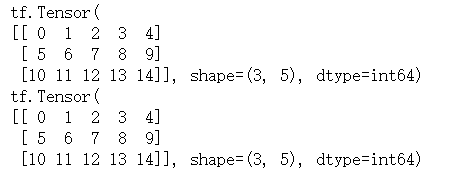3. Tensor的属性

• 形状 shape：

Tensor31.shape

• 数据类型 dtype：

Tensor31.dtype

• 对应的 NumPy 数组：

Tensor31.numpy()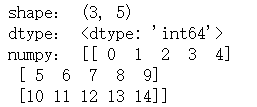4. 新建特殊常量张量的方法

• tf.zeros ：新建指定形状且全为 0 的常量 Tensor
• tf.zeros_like ：参考某个Tensor的形状，新建全为 0 的常量 Tensor
• tf.ones ：新建指定形状且全为 1 的常量 Tensor
• tf.ones_like ：参考某种形状，新建全为 1 的常量 Tensor
• tf.fill ：新建一个指定形状且全为某个标量值的常量 Tensor
# tf.zeros(shape, dtype=tf.float32, name=None)
Tensor21 = tf.zeros([3, 3])
# tf.zeros_like(input, dtype=None, name=None)
Tensor22 = tf.zeros_like(Tensor21) # 输入为Tensor， 意为与Tensor形状一致，且值全为0的常量Tensor
# tf.fill(dims, value, name=None)
Tensor23 = tf.fill([3, 3], 2)

print(Tensor21)
print(Tensor22)
print(Tensor23)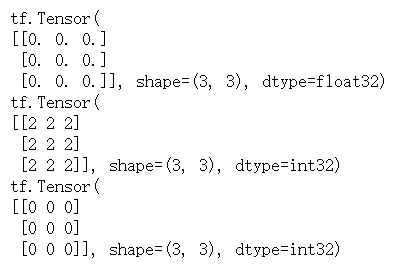5. 生成随机张量：

• 均匀分布的随机数

# 均匀分布在区间[minval, maxval]的随机数
tf.random.uniform(shape=[2,3], minval=0, maxval=10)

• 生成正态分布的随机数

# 生成正态分布的随机数。默认均值为0、标准差为1
tf.random.normal(shape=[2,3], mean=10, stddev=1)

• 生成截断式正态分布的随机数

# 生成截断式正态分布的随机数
tf.random.truncated_normal(shape=[2,3], mean=10, stddev=1)
# 如果生成的数据在(μ-2δ, μ+2δ)之外，则重新进行生成，保证生成值在均值附近。
# δ：均值，δ：标准差

6. 常用方法

• 强制类型转换并不会改变原来的类型，而是返回一个新的张量

# tf.cast(x, dtype, name=None)
print(Tensor41.dtype)
ts1 = tf.cast(Tensor41, dtype=tf.int32)

print(Tensor41.dtype)
print(ts1.dtype)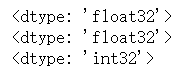• 求张量维度上元素的最大/小值

# tf.reduce_min(input_tensor, axis=None, keepdims=False, name=None)
print(Tensor41)

print(tf.reduce_min(Tensor41))
# tf.reduce_max(input_tensor, axis=None, keepdims=False, name=None)
print(tf.reduce_max(Tensor41))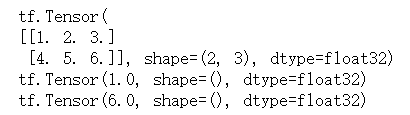7. 其他方法

• tf.linspace()

创建一个等间隔序列。

• tf.range()

创建一个数字序列。

# tf.linspace(start, stop, num, name=None)
tf.linspace(2.0, 6.0, 3)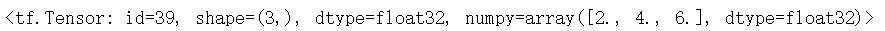## 2. 操作(operation)

​ TensorFlow 里有大量的 操作 （Operation），使得我们可以将已有的张量进行运算后得到新的张量。

​ 定义如下Tensor

Tensor41 = tf.constant(tf.linspace(1.0, 6.0, 6), shape=(2,3))
Tensor42 = tf.constant(tf.linspace(1.0, 9.0, 9), shape=(3,3))
Tensor43 = tf.constant(tf.linspace(7.0, 12.0, 6), shape=(2,3))
print(Tensor41)
print(Tensor42)
print(Tensor43)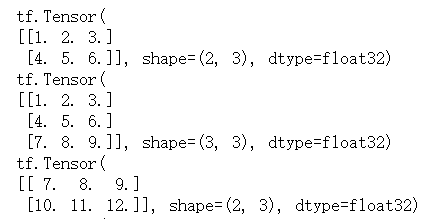### 2.1 四则运算

# add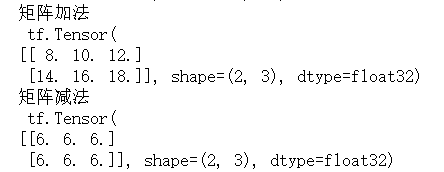# subtract
print(tf.subtract(Tensor43, Tensor41))
# multiply
print("数值 x 矩阵\n", tf.multiply(10, Tensor41))
print("矩阵 x 矩阵\n", tf.multiply(Tensor43, Tensor41))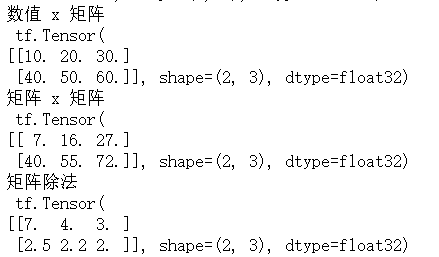### 2.2 平方、次方、开方

# 定义张量
Tensor5 = tf.fill([3,3], 3)

• 平方

# 平方。tf.square(x, name=None)
print(tf.square(Tensor5))
# 等价于
print(tf.multiply(Tensor5, Tensor5))
print(tf.pow(Tensor5, 2))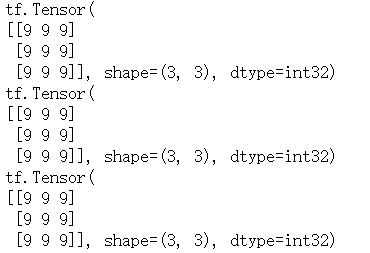• n次方

# n次方。tf.pow(x, y, name=None)
print(tf.pow(Tensor5, 2))

• 开平方

# 开平方。tf.sqrt(x, name=None)
Tensor51 = tf.fill([3,3], 9.0)

print(Tensor51)
print(tf.sqrt(Tensor51))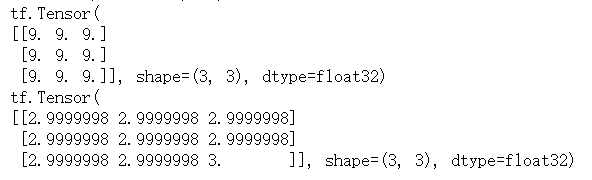### 2.3 点乘

# matmul
Tensor4_mat = tf.matmul(Tensor41, Tensor42)
print(Tensor4_mul)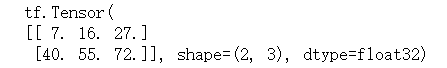### 2.4 矩阵转置

Tensor41 = tf.constant(line[0:5], dtype=float, shape=[1, 5])
# Tensor42 = tf.linalg.matrix_transpose(Tensor41) # 报错
Tensor42 = tf.transpose(Tensor41)
print("矩阵转置\n")
print(Tensor41)
print(Tensor42)


## 3. 常用函数

### 3.1 特征--标签配对

# tf.data.Dataset.from_tensor_slices( (tensor_label, tensor_feature) )。

X = tf.constant([12,21,22,34])
y = tf.constant([0,1,1,0])
dataset = tf.data.Dataset.from_tensor_slices((X, y))
print(dataset)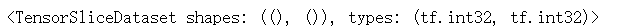for e in dataset:
print(e)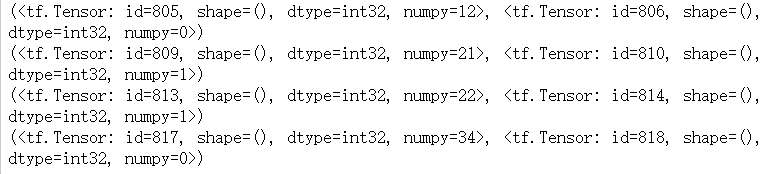### 3.2 自动求导

1. 微分概念：

TensorFlow 中，你可以使用 tf.GradientTape 跟踪全部运算过程，以便在必要的时候计算梯度。然后用tf.GradientTape().gradient 进行求导计算。

with tf.GradientTape() as tape: # 追踪梯度
w = tf.Variable([2.0])
loss = tf.pow(w, 3)

# loss 对 w 求导
# 求导结果为：3*w*w => 12
# 输出grap，与我们计算的值一致。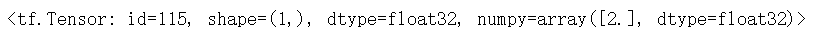### 3.3 枚举函数

enumerate 函数可将一个可遍历的数据对象(如列表、元组或字符串)

lst = ['aa', 'bb', 'cc']
for index, value in enumerate(lst):
print("%s : %s" %(index,value))


### 3.4 独热编码

# 如鸢尾花数据，三分类。
y = tf.constant([1,1,0,2])
classes = 3
y_one_hot = tf.one_hot(y, classes)
print(y_one_hot)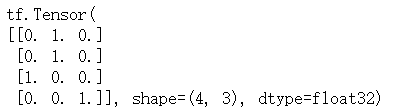0 ==> 1 0 0

1 ==> 0 1 0

2 ==> 0 0 1

• 将每一个数转换成长度为x位、值全为 0 的二进制数
• 第n位的 0 转换成1。(位数从0到x-1)

### 3.5 归一化指数函数

softmax函数可以使n分类的n个输出y0,y1...中，各个输出的概率值。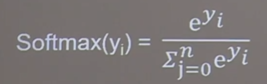### 3.6 自减函数

• 赋值操作，更新参数的值并返回。

• 调用assign_sub前，先用 tf.Variable 定义变量w为可训练（可自更新）。

w = tf.Variable(tf.constant(4.0))
w.assign_sub(1)
# 相当于： w -= 1
w.numpy()


### 3.7 最大值索引

tf.argmax(张量名, axis=操作轴)

## 4. 常用模块

• tf.：包含了张量定义，变换等常用函数和类。
• tf.data：输入数据处理模块，提供了像 tf.data.Dataset 等类用于封装输入数据，指定批量大小等。
• tf.image：图像处理模块，提供了像图像裁剪，变换，编码，解码等类。
• tf.keras：原 Keras 框架高阶 API。包含原 tf.layers 中高阶神经网络层。
• tf.linalg：线性代数模块，提供了大量线性代数计算方法和类。
• tf.losses：损失函数模块，用于方便神经网络定义损失函数。
• tf.math：数学计算模块，提供了大量数学计算函数。
• tf.saved_model：模型保存模块，可用于模型的保存和恢复。
• tf.train：提供用于训练的组件，例如优化器，学习率衰减策略等。
• tf.nn：提供用于构建神经网络的底层函数，以帮助实现深度神经网络各类功能层。
• tf.estimator：高阶 API，提供了预创建的 Estimator 或自定义组件。
posted @ 2020-11-04 21:41  linlk  阅读(66)  评论(0编辑  收藏  举报